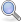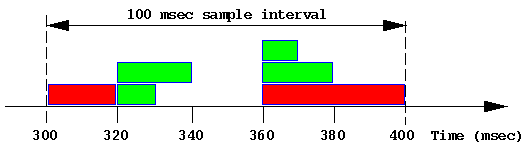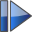# How to understand measures of disk performance

 ```Tools pmchart sar ```

This chapter of the Performance Co-Pilot tutorial provides some hints on how to interpret and understand the various measures of disk performance.

For an explanation of Performance Co-Pilot terms and acronyms, consult the PCP glossary.

 Reconciling sar -d and PCP disk performance metrics

Both sar and Performance Co-Pilot (PCP) use a common collection of disk performance instrumentation from the block layer in the kernel, however the disk performance metrics provided by sar and PCP differ in their derivation and semantics.  This document is an attempt to explain these differences.

It is convenient to define the ``response time'' to be the time to complete a disk operation as the sum of the time spent:

• entering the read() or write() system call and set up for an I/O operation (time here is CPU bound and is assumed to be negligible per I/O)
• in a queue of pending requests waiting to be handed to the device controller (the ``queue time'')
• the time between the request being handed to the device controller and the end of transfer interrupt (the ``(device) service time''), typically composed of delays due to request scheduling at the controller, bus arbitration, possible seek time, rotational latency, data transfer, etc.
• time to process the end of transfer interrupt, housekeeping at the end of an I/O operation and return from the read() or write() system call (time here is CPU bound and also assumed to be negligible per I/O)

Note that while the CPU time per I/O is assumed to be small in relationship to the times involving operations at the device level, when the system-wide I/O rate is high (and it could be tens of thousands of I/Os per second on a very large configuration), the aggregate CPU demand to support this I/O activity may be significant.

The kernel agents for PCP export the following metrics for each disk spindle:

Table 1: Raw PCP disk metrics
Metric Units Semantics
disk.dev.write number running total of write I/O requests since boot time
disk.dev.total number running total of I/O requests since boot time, equals disk.dev.read + disk.dev.write
disk.dev.blkread number running total of data read since boot time in units of 512-byte blocks
disk.dev.blkwrite number running total of data written since boot time in units of 512-byte blocks
disk.dev.blktotal number running total of data read or written since boot time in units of 512-bytes, equals disk.dev.blkread + disk.dev.blkwrite
disk.dev.read_bytes Kbytes running total of data read since boot time in units of Kbytes
disk.dev.write_bytes Kbytes running total of data written since boot time in units of Kbytes
disk.dev.bytes Kbytes running total of data read or written since boot time in units of Kbytes, equals disk.dev.read_bytes + disk.dev.write_bytes
disk.dev.active milliseconds running total (milliseconds since boot time) of time this device has been busy servicing at least one I/O request
disk.dev.response milliseconds running total (milliseconds since boot time) of the response time for all completed I/O requests

These metrics are all "counters" so when displayed with most PCP tools, they are sampled periodically and the differences between consecutive values converted to rates or time utilization over the sample interval as follows:

Table 2: PCP disk metrics as reported by most PCP tools
Metric Units Semantics
disk.dev.write number per second write I/O requests per second (or write IOPS)
disk.dev.total number per second I/O requests per second (or IOPS)
disk.dev.blkwrite number per second 2 * (Kbytes written per second)
disk.dev.blktotal number per second 2 * (Kbytes read or written per second)
disk.dev.write_bytes Kbytes per second Kbytes written per second
disk.dev.bytes Kbytes per second Kbytes read or written per second
disk.dev.active time utilization fraction of time device was "busy" over the sample interval (either in the range 0.0-1.0 or expressed as a percentage in the rance 0-100); in this context "busy" means servicing one or more I/O requests
disk.dev.response time utilization time average of the response time over the interval; this is a slightly strange metric in that values larger than 1.0 (or 100%) imply either device saturation, or controller saturation or a very ``bursty'' request arrival pattern -- in isolation there is no sensible interpretation of the rate converted value this metric

The sar metrics avque, avwait and avserv are subject to widespread misinterpretation, and so warrant some special explanation. They may be understood with the aid of a simple illustrative example. Consider the following snapshot of disk activity in which the response time has been simplified to be a multiple of 10 milliseconds for each I/O operation over a 100 millisecond sample interval (this is an unlikely simplification, but makes the arithmetic easier).Each green block represents a 4 Kbyte read. Each red block represents a 16Kbyte write.

avque

The stochastic average of the "queue" length sampled just before each I/O is complete, where ``queue'' here includes those requests in the queue and those being serviced by the device controller. Unfortunately the stochastic average of a queue length is not the same as the more commonly understood temporal or time average of a queue length.

In the table below, R is the contribution to the sum of the response times, Qs is the contribution to the sum of the queue length used to compute the stochastic average and Qt is the contribution to the sum of the queue length × time used to compute the temporal average.

Time
(msec)
Event R
(msec)
Qs Qt
(msec)
300 Start I/O #1 (write)
320 End I/O #1 20 1 1×20
330 End I/O #2 10 2 2×10
340 End I/O #3 20 1 1×10
360 Start I/O #4 (write)
370 End I/O #6 10 3 3×10
380 End I/O #5 20 2 2×10
400 End I/O #4 40 1 1×20

The (stochastic) average response time is sum(R) / 6 = 120 / 6 = 20 msec.

The stochastic average of the queue length is sum(Qs) / 6 = 10 / 6 = 1.67.

The temporal average of the queue length is sum(Qt) / 100 = 120 / 100 = 1.20.

Even in this simple example, the two methods for computing the "average" queue length produce different answers. As the inter-arrival rate for I/O requests becomes more variable, and particularly when many I/O requests are issued in a short period of time followed by a period of quiescence, the two methods produce radically different results.

For example if the idle period in the example above was 420 msec rather than 20 msec, then the stochastic average would remain unchanged at 1.67, but the temporal average would fall to 120/500 = 0.24 ... given that this disk is now idle for 420/500 = 84% of the time one can see how misleading the stochastic average can be. Unfortunately many disks are subject to exactly this pattern of short bursts when many I/Os are enqueued, followed by long periods of comparative calm (consider flushing dirty blocks by bdflush in IRIX or the DBWR process in Oracle). Under these circumstances, avque as reported by sar can be very misleading.

avserv

Because multiple operations may be processed by the controller at the same time, and the order of completion is not necessarily the same as the order of dispatch, the notion of individual service time is difficult (if not impossible) to measure. Rather, sar approximates using the total time the disk was busy processing at least one request divided by the number of completed requests.

In the example above this translates to busy for 80 msec, in which time 6 I/Os were completed, so the average service time is 13.33 msec.

avwait

For reasons similar to those applying to avserv the average time spent waiting cannot be split between waiting in the queue of requests to be sent to the controller and waiting at the controller while some other concurrent request is being processed. So sar computes the total time spent waiting as the total response time minus the total service time, and then averages over the number of completed requests.

In the example above this translates to a total waiting time of 120 msec - 80 msec, in which time 6 I/Os were completed, so the average waiting time is 6.67 msec.

When run with a -d option, sar reports the following for each disk spindle:

Table 3: PCP and sar metric equivalents
Metric Units PCP equivalent
(in terms of the rate converted metrics in Table 2)
%busy percent 100 * disk.dev.active
avque I/O operations N/A (see above)
r+w/s I/Os per second disk.dev.total
blks/s 512-byte blocks per second disk.dev.blktotal
w/s write I/Os per second disk.dev.write
wblks/s 512-byte blocks written per second disk.dev.blkwrite
avwait milliseconds 1000 * (disk.dev.response - disk.dev.active) / disk.dev.total
avserv milliseconds 1000 * disk.dev.active / disk.dev.total

The table below shows how the PCP tools and sar would report the disk performance over the 100 millisecond interval from the example above:

Table 3: Illustrative values and calculations
Rate converted PCP metric
(like in Table 2)
sar metrics Explanation
disk.dev.write w/s 2 writes in 100 msec = 20 writes per second
disk.dev.total r+w/s 4 reads + 2 write in 100 msec = 60 I/Os per second
disk.dev.blkread N/A 4 * 4 Kbytes = 32 blocks in 100 msec = 320 blocks read per second
disk.dev.blkwrite wblks/s 2 * 16 Kbytes = 64 blocks in 100 msec = 640 blocks written per second
disk.dev.blktotal blks/s 96 blocks in 100 msec = 960 blocks per second
disk.dev.read_bytes N/A 4 * 4 Kbytes = 16 Kbytes in 100 msec = 160 Kbytes per second
disk.dev.write_bytes N/A 2 * 16 Kbytes = 32 Kbytes in 100 msec = 320 Kbytes per second
disk.dev.bytes N/A 48 Kbytes in 100 msec = 480 Kbytes per second
disk.dev.active %busy 80 msec active in 100 msec = 0.8 or 80%
disk.dev.response N/A Disregard (see comments in Table 2)
N/A avque 1.67 requests (see derivation above)
N/A avwait 6.67 msec (see derivation above)
N/A avserv 13.33 msec (see derivation above)

In practice many of these metrics are of little use. Fortunately the most common performance problems related to disks can be identified quite simply as follows:

Device saturation
Occurs when disk.dev.active is close to 1.0 (which is the same as %busy is close to 100%).
Device throughput
Use disk.dev.bytes (or blks/s divided by 2 to produce Kbytes per second)
The peak value depends on the bus and disk characteristics, and is subject to significant variation depending on the distribution, size and type of requests. Fortunately in many environments the peak value does not change over time, so once established, monitoring thresholds tend to remain valid.
For some disks (and RAID devices in particular) writes may be slower than reads. The ratio of disk.dev.write to disk.dev.total (or w/s to r+w/s) indicates the fraction of I/O requests that are writes.

In terms of the available instrumentation from the IRIX kernel, one potentially useful metric would be the stochastic average of the response time per completed I/O operation, which in the sample above would be 20 msec. Unfortunately no performance tool reports this directly.

• For sar, this metric is the sum of avwait and avserv.

• The common PCP tools only support temporal rate conversion for counters, however the stochastic average of the response time can be computed with the PCP inference engine (pmie) using an expression of the form:
```avg_resp = 1000 * disk.dev.response / disk.dev.total;
```

 A real example

Consider this data from sar -d with a 10 minute update interval:

``` device %busy  avque  r+w/s  blks/s    w/s wblks/s  avwait  avserv
dks0d2    34   12.8     32     988     29     874   123.1    10.5
dks0d5    34   12.5     33    1006     29     891   119.0    10.4
```

At first impression, queue lengths of 12-13 requests and wait time of 120msec looks pretty bad.

But further investigation is warranted ...

• most of the I/Os are writes (58 of 65 I/Os per second)
• average I/Os are (874+891)*512/(29+29) = 15580 bytes ... close to default 16K filesystem block size
• to sustain (874+891)*512 = 903680 bytes of write throughput per second for at least 10 minutes you are doing a lot of file writes
• the disks are not unduly busy at 34% utilization
• consider what happens when bdflush, pdflush and friends run ... lets make some simplifying assumptions to make the arithmetic easy
• we are dirtying (writing) 60 x 16 Kbyte pages (983040 bytes) per second
• flushing goes off every 10 seconds, but the page cache is scanned in something under 10 msec
• to keep up, each flush must push out 600 pages
• I/O is balanced across 2 disks
• disk service time is 10 msec per I/O
• after the flushing code has scanned the page cache, all 300 writes per disk are on the queue before the first one is done (this is what skews the wait time and queue lengths)
• disk utilization is 300 * 10 / (10 * 1000) = 0.3 = 30%
• the stochastic average wait time is (0 + 10 + 20 + ... + 2990) / 300 > = 150 msec
• time to empty the queue after a flush is 3 seconds
• the temporal average queue length is 0 * 7/10 + 150 * 3/10 = 45

The complicating issue here is that the I/O demand is very bursty and this is what skews the "average" measures.

In this case, the I/O is probably asynchronous with respect to the process(es) doing the writing. Under these circumstances, performance is unlikely to improve dramatically if the aggregate I/O bandwidth was increased (e.g. by spreading the writes across more disk spindles).

However if the I/O is synchronous (e.g. it it was read dominated, or the I/O was to a raw disk), then more I/O would reduce application running time.

There are also hybrid scenarios in which a small number of synchronous reads are seriously slowed down during the bursts of asynchronous writes. In the example above, a read could have the misfortune of being queued behind 300 writes (or delayed for 3 seconds).

 Beware of Wait I/O

PCP (and sar and osview and ...) all report CPU utilization broken down into:

• user
• system (sys, intr)
• idle
• wait (for file system I/O, graphics, physical I/O and swap I/O)

Because I/O does not "belong" to any processor (and in some cases may not "belong" to any current process), a CPU that is "waiting for I/O" is more accurately described as an "idle CPU while at least one I/O is outstanding".

Anomalous Wait I/O time occurs under light load when a small number of processes are waiting for I/O but many CPUs are otherwise idle, but appear in the "Wait for I/O" state. When the number of CPUs increases to 30, 60 or 120 then 1 process doing I/O can make all of the CPUs except 1 look like they are all waiting for I/O, but clearly no amount of I/O bandwidth increase is going to make any difference to these CPUs. And if that one process is doing asynchronous I/O and not blocking, then additional I/O bandwidth will not make it run faster either.Using pmchart to display concurrent disk and CPU activity (aggregated over all CPUs and all disks respectively). ``` \$ source /etc/pcp.conf \$ tar xzf \$PCP_DEMOS_DIR/tutorials/diskperf.tgz \$ pmchart -t 2sec -O -0sec -a diskperf/waitio -c diskperf/waitio.view ``` The system has 4 CPUs, several disks and only 1 process really doing I/O. Note that over time: in the top chart as the CPU user (blue) and system (red) time increases, the Wait I/O (pale blue) time decreases from the bottom chart, the I/O rate is pretty constant throughout in the bursts where the I/O rate falls, the Wait I/O time becomes CPU idle (green) time